### 贪心算法思想

​ 1.贪心算法（又称贪婪算法）是指，在对问题求解时，总是做出在当前看来是最好的选择。也就是说，不从整体最优上加以考虑，他所做出的是在某种意义上的局部最优解。
​ 2.贪心选择是指所求问题的整体最优解可以通过一系列局部最优的选择，即贪心选择来达到。这是贪心算法可行的第一个基本要素。
​ 3.当一个问题的最优解包含其子问题的最优解时，称此问题具有最优子结构性质。运用贪心策略在每一次转化时都取得了最优解。问题的最优子结构性质是该问题可用贪心算法求解的关键特征。贪心算法的每一次操作都对结果产生直接影响。贪心算法对每个子问题的解决方案都做出选择，不能回退。
​ 4.贪心算法的基本思路是从问题的某一个初始解出发一步一步地进行，根据某个优化测度，每一步都要确保能获得局部最优解。每一步只考虑一个数据，他的选取应该满足局部优化的条件。若下一个数据和部分最优解连在一起不再是可行解时，就不把该数据添加到部分解中，直到把所有数据枚举完，或者不能再添加算法停止。
​ 5.实际上，贪心算法适用的情贪心算法(贪婪算法)况很少。一般对一个问题分析是否适用于贪心算法，可以先选择该问题下的几个实际数据进行分析，就可以做出判断。

1.不能保证求得的最后解是最佳的
2.不能用来求最大值或最小值的问题
3.只能求满足某些约束条件的可行解的范围

### 选择排序

​ 我们熟知的选择排序，其实采用的即为贪心策略。

​ 它所采用的贪心策略即为每次从未排序的数据中选取最小值，并把最小值放在未排序数据的起始位置，直到未排序的数据为0，则结束排序。

```import java.util.Arrays;
/**
* 选择排序
*/
public class TestDemo {
public static void swap(int[] arr, int i, int j){
int tmp = arr[i];
arr[i] = arr[j];
arr[j] = tmp;
}
public static void selectSort(int[] arr){
//i: 未排序数据的起始位置
for(int i = 0; i < arr.length; ++i)
{
int minIdx = i;
//从所有未排序的数据中找最小值的索引
for(int j = i + 1; j < arr.length; ++j){
if(arr[j] < arr[minIdx])
minIdx = j;
}
swap(arr, minIdx, i);
}
}
public static void main(String[] args) {
int[] arr = {10, 3, 6, 0, 8};
selectSort(arr);
System.out.println(Arrays.toString(arr));
}
}```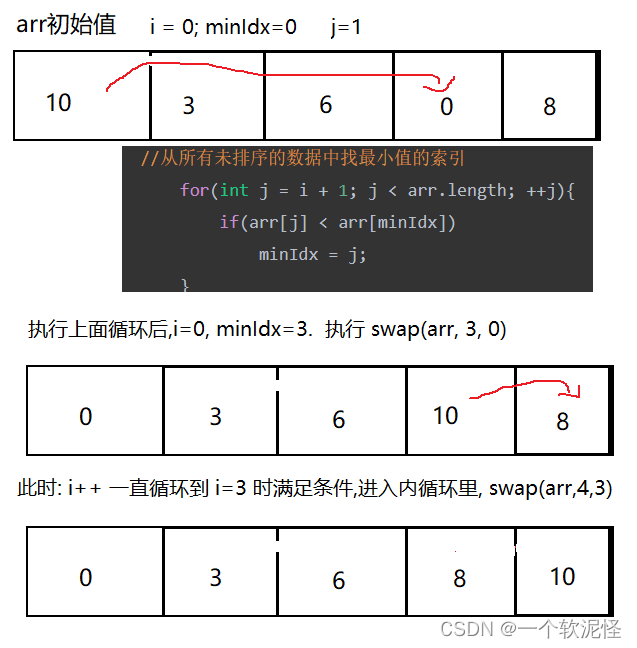### 平衡字符串

https://leetcode-cn.com/problems/split-a-string-in-balanced-strings/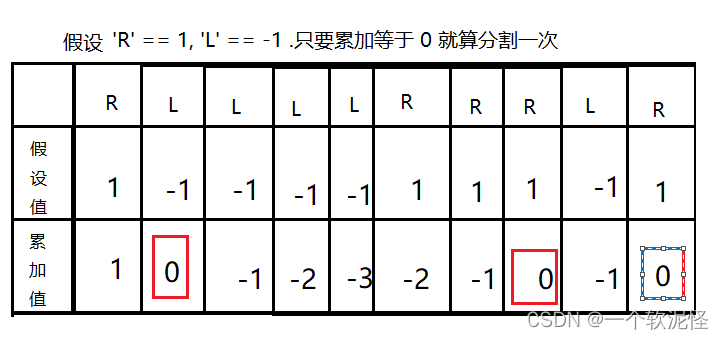```class Solution {
public int balancedStringSplit(String s) {
int cnt = 0;
int balance = 0;
for(int i = 0; i < s.length(); i++){
if(s.charAt(i) == 'L')
balance--;
if(s.charAt(i) == 'R')
balance++;
if(balance == 0)
cnt++;
}
return cnt;
}
}```

### 买卖股票的最佳时机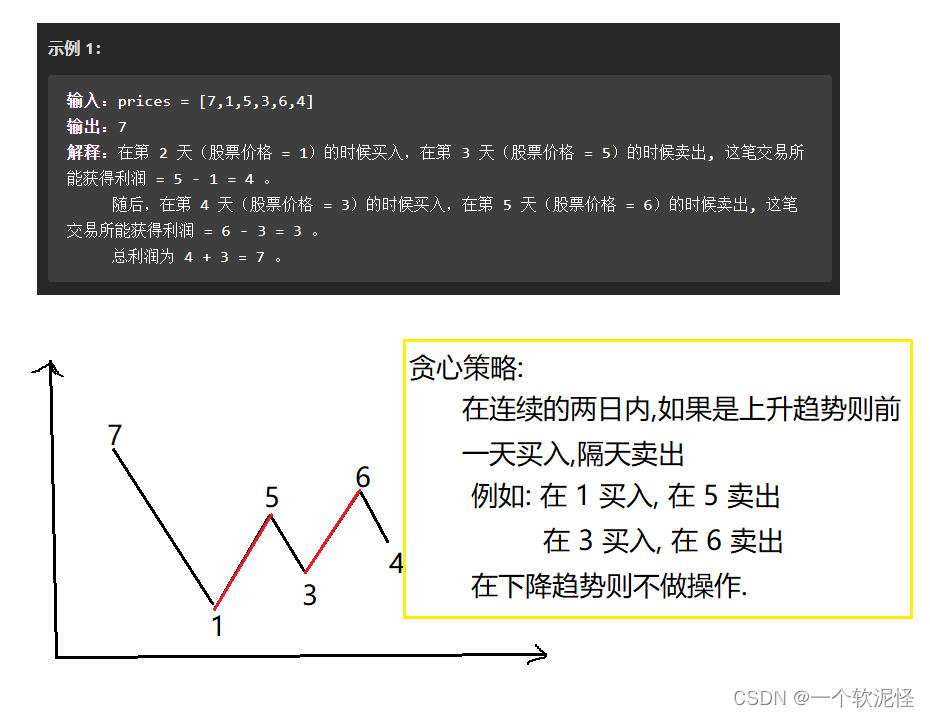```class Solution {
public int maxProfit(int[] prices) {
int ret = 0;
for(int i = 1; i < prices.length; ++i)
{
int curProfit = prices[i] - prices[i - 1];
if(curProfit > 0)
ret += curProfit;
}
return ret;
}
}```

### 跳跃游戏

https://leetcode-cn.com/problems/jump-game/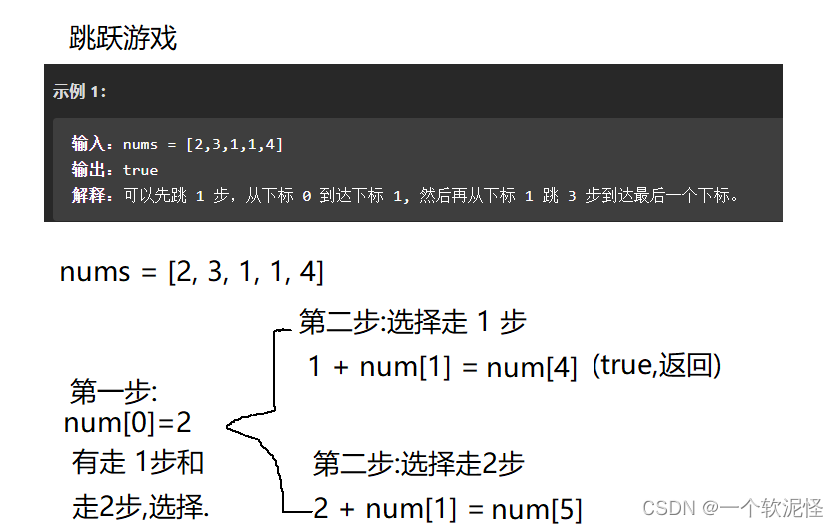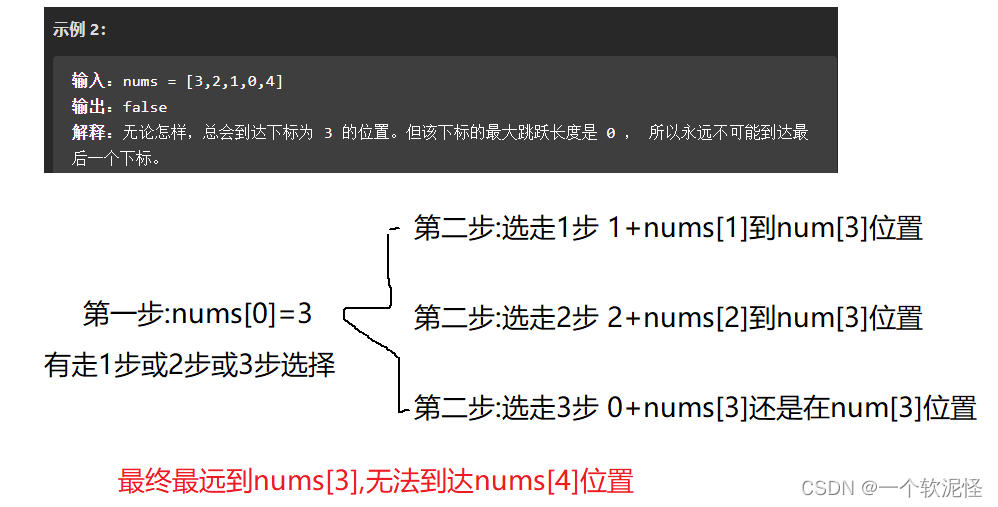```/**
* 跳跃位置
*/
public class TestDemo3 {
public class Solution {
public boolean canJump(int[] nums) {
int pos = nums.length - 1;
int rightmost = 0;
for (int i = 0; i <= pos; ++i) {
//如果可以到达当前位置，则更新最大
if (i <= rightmost) {
//每次更新最大的位置
rightmost = Math.max(rightmost, i + nums[i]);
//如果可以到达最后一个位置，则直接返回
if (rightmost >= pos) {
return true;
}
}
}
return false;
}
}
}```

### 钱币找零

```import java.util.Scanner;
/**
* 纸币找零
*/
public class TestDemo4 {
public static int solve(int money, int[][] moneyCount)
{
int num = 0;
//首先选择最大面值的纸币
for (int i = moneyCount.length - 1; i >= 0; i--)
{
//需要的当前面值与面值数量取最小
int c = Math.min(money / moneyCount[i], moneyCount[i]);
money = money - c * moneyCount[i];
num += c;
}
if (money > 0)
num = -1;
return num;
}
public static void main(String[] args) {
//存放纸币与数量: first:纸币，second:数量
int[][] moneyCount = { { 1, 3 }, { 2, 1 }, { 5, 4 }, { 10, 3 }, { 20, 0 }
,{50, 1}, { 100, 10 } };
Scanner scanner = new Scanner(System.in);
int money;
System.out.println("请输入要支付的钱");
money = scanner.nextInt();
int res = solve(money, moneyCount);
if (res != -1)
System.out.println(res);
else
System.out.println("No");
}
}```

### 多机器调度问题

1<=m<=100），接下来的一行是n个整数ti（1<=t<=100)。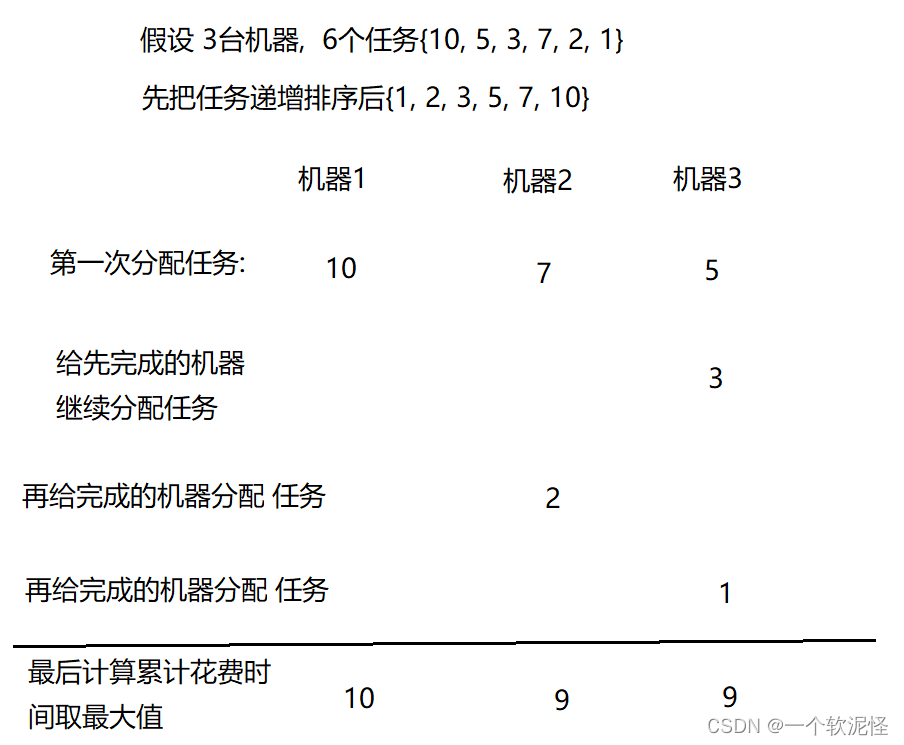```import java.util.Arrays;
import java.util.Scanner;
/**
*多机器调度问题
*/
public class TestDemo5 {
public static int findMax(int[] machines){
int ret = machines;
for (int cur : machines)
{
if (ret < cur)
ret = cur;
}
return ret;
}
private static int greedStrategy(int[] taskTime, int m) {
// 按作业时间从小到大排序
int[] machines = new int[m];
// 作业数如果小于机器数，直接返回最大的作业时间
if (n <= m) {
} else {
for (int i = n-1; i >= 0; --i) {
// 找到最先结束的机器,分配新的任务
int finish = 0;
//首先假设用第一个机器处理
int machineTime = machines[finish];
// 从剩余机器中选择作业时间最小的
for (int j = 1; j < m; j++) {
if (machineTime > machines[j]) {
finish = j;
machineTime = machines[j];
}
}
// 将新的任务分配给最先结束的机器执行
}
// 从所有机器中选出最后执行完作业的机器
return findMax(machines);
}
}
public static void main(String[] args) {
int n, m;
System.out.println("请输入作业个数和机器数");
Scanner scanner = new Scanner(System.in);
n = scanner.nextInt();
m = scanner.nextInt();
System.out.println("输入任务的时间");
for (int i = 0; i < n; ++i){
}
}
}```

### 举办活动数量最多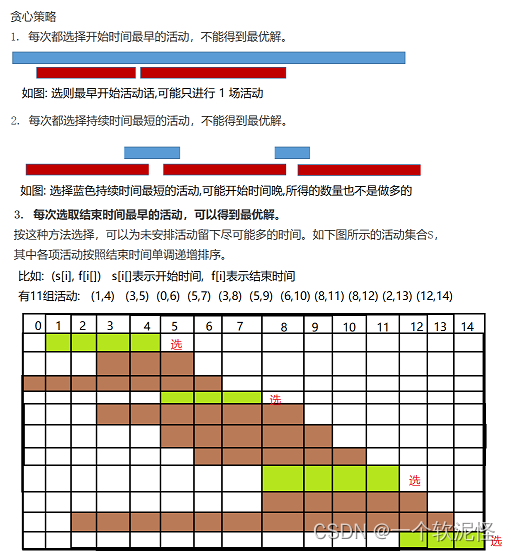```import java.util.Arrays;
import java.util.Comparator;
import java.util.Scanner;

/**
* 举办活动数量最多的
*/
class myComparator implements Comparator<int[]> {
//按起点递增排序
public int compare(int[] a, int[] b) {
return a - b;
}
}
public class TestDemo6 {
public static int greedyActivitySelector(int[][] act)
{
//贪婪策略：每次选择最早结束的活动
int num = 1, i = 0;
for (int j = 1; j < act.length; j++)
{
if (act[j] >= act[i])
{
i = j;
num++;
}
}
return num;
}
public static void main(String[] args) {
Scanner scanner = new Scanner(System.in);
int number = scanner.nextInt();
int[][] act = new int[number];
for (int i = 0; i < act.length; ++i)
{
act[i] = scanner.nextInt();
act[i] = scanner.nextInt();
}
//按照活动截止时间从小到大排序
Arrays.sort(act, new myComparator());
int ret = greedyActivitySelector(act);
System.out.println(ret);
}
}```

### 无重叠区间

https://leetcode-cn.com/problems/non-overlapping-intervals/

```class myComparator implements Comparator<int[]> {
//按最早结束递增排序
public int compare(int[] a, int[] b) {
return a - b;
}
}
class Solution {
public int eraseOverlapIntervals(int[][] intervals) {
if (intervals.length == 0) {
return 0;
}
//按照结束进行递增排序
Arrays.sort(intervals, new myComparator());
//贪婪策略：每次选择最早结束的活动
int num = 1, i = 0;
for (int j = 1; j < intervals.length; j++)
{
if (intervals[j] >= intervals[i])
{
i = j;
num++;
}
}
return intervals.length - num;
}
}```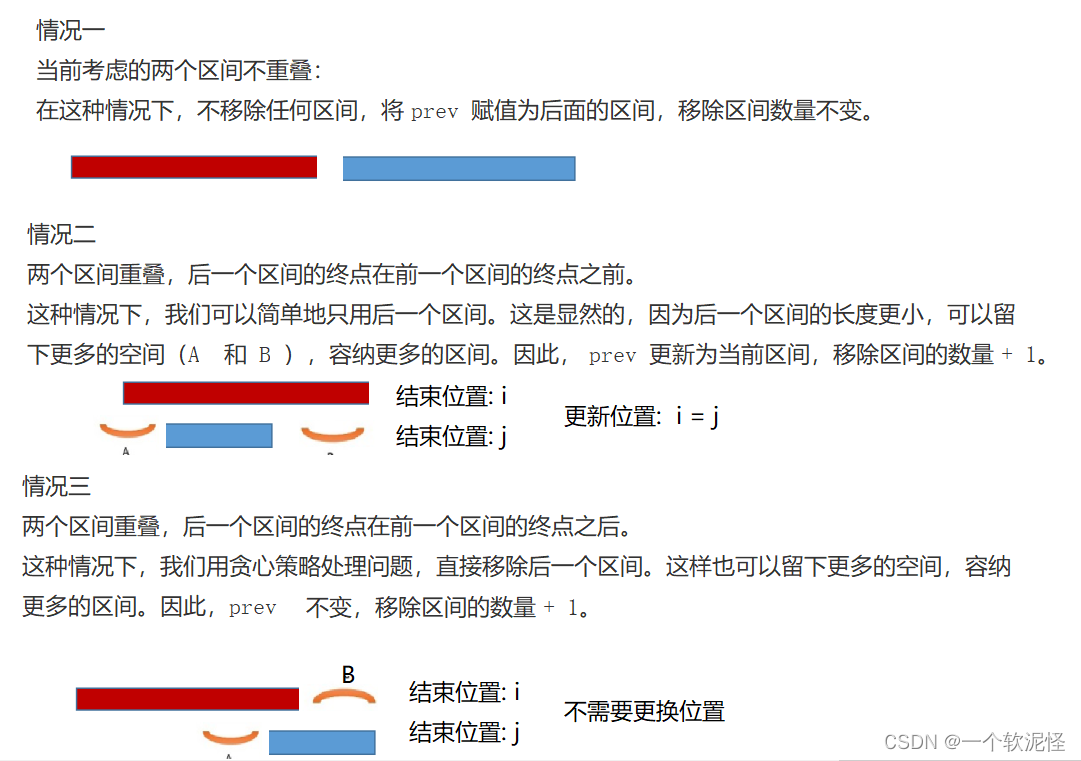```class myComparator implements Comparator<int[]> {
//按起点递增排序
public int compare(int[] a, int[] b) {
return a - b;
}
}
class Solution {
public int eraseOverlapIntervals(int[][] intervals) {
if (intervals.length == 0) {
return 0;
}
//按照起始进行递增排序
Arrays.sort(intervals, new myComparator());
//贪婪策略：每次选择最早结束的活动
int num = 0, i = 0;
for (int j = 1; j < intervals.length; j++)
{
if (intervals[j] >= intervals[i])
{
//下一场活动开始时间大于等于上一场活动结束时间
//不需要更新
i = j;
}else{
if(intervals[j] < intervals[i])
{
//下一场活动结束时间小于上一场活动结束时间
//更新位置,把上一场删除
i = j;
}
++num;
}
}
return num;
}
}```